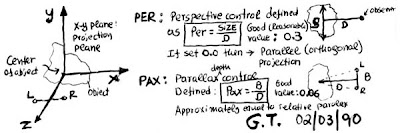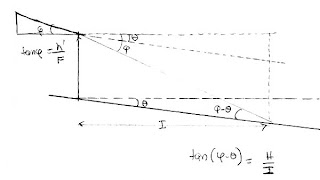## Friday, March 7, 2008

### Stereo DrawingsBack in 1988-1990 I wrote a program in “C” (that’s a programming language), to create stereoscopic drawings. It worked like this: The program will read a data file with the three coordinates of an object in space (X, Y, Z) plus simple instructions for plotting them, and then it would create a stereo pair. A fancy (for 1990 computer technology) interface allowed the user to rotate the image in space, while viewing it in 3d.

This was a nice learning experience for me and took quite a bit of time. See here some examples of the drawings I produced (click to enlarge) and also of the keyboard layout that allowed me to rotate, translate (even in the z-direction, i.e. affecting the stereo window), magnify the image, and also change the two important variables, perspective and parallax.

I am amazed that I did this work 18 years ago and then abandoned it. I am now feeling an urge to resume this, but what tools to use today? The reason I want to resume this work is that I want to study some interesting situations, like “keystoning” using simple drawings instead of actually trying to take pictures.The program did a lot of things, like scaling, centering, rotating, etc., but the most important operation for 3d purposes is the projection of the point on the “plane of the screen”. Here is how this goes (see figure on the left):

We start with a point in space with coordinates (Xo, Yo, Zo). The observer is sitting at the point Z in the z-axis. If from this point Z we project this point to the projection plane (x, y), we get these projection coordinates: (Xc, Yc). We then move the observer to the Right and Left by B/2 each time (B is the stereo base) and project. Only the x-projections change. In the end, we get two pairs of points (XR, YR=Yc), (XL, YL=Yc). These are the coordinates of our starting point in the right and left projections.I soon realized that there are two variables involved: One is the stereo base (B), which controls the stereoscopic deviation in the pair. This is clear. The other variable is not so obvious: For a given object, it is possible to come closer and photograph it with a short FL lens, or stay back and photograph it with the long FL lens. The difference between these two situations is the perspective. So I decided to use two variables in my program:

- PAX (Parallax) defined as B/D
- PER (Perspective) defined as S/D

B is the Stereo Base, S is the size of the object, D is the distance of the “camera” from the object.

The Parallax value is what I have called in previous postings “convergence ratio”, B/I in my current terminology. The Perspective variable is inversely proportional to the distance. It is related to the angle of view that the lens sees, which is also related to the size of the film and inversely related to the focal length. We can call this variable the “field of view ratio”.

It is interesting that I note as reasonable values 0.3 for PER (a “normal lens” has a ratio closer to 1. A ratio of 0.3 corresponds to lens of focal length between 80 and 120mm for 35mm film(depending on how you define the film angle of view, horizontal, vertical, or diagonal – the diagonal dimension is normally considered, but in stereo the horizontal is more appropriate, but should this be 36mm or maybe 28mm-7p or 21mm-5p?). So my choice of 0.3 is definitely conservative, meaning that it leads to milder perspective changes.I also note that 0.06 is a good convergence ratio. Written as a ratio, this is 1/17, which is stronger than the 1/30 rule of thumb for normal stereo photography, but more in line with close-up/macro stereo photography.

It is interesting to study the formulas that give the coordinates of the projected points. There is a variable (which I called M because it looks like a magnification factor since it affects both variables the same degree.) This variable is not constant but it depends on distance (z) only. This expresses the perspective. Object points close to the camera, project further away, i.e. they look larger. The true stereo part of the coordinates depends also on the distance. As expected, objects closer to the camera have more depth. As we have discussed, distance affects all three metric aspects of the stereo image: Size, perspective, and depth.

Two interesting situations: If we set PAX = 0 (B = 0), there is no stereo! The two projections will be identical. The 2d images will have perspective but it will be flat. If we set PER = 0 we can still create a stereo pair, but there will be no perspective. This is known as “orthogonal projection”. Now, to have zero perspective we must be far away from the object, but then how do we have depth? By increasing the stereo base. The bottom line is that by changing our two variables we can create stereoscopic drawings that have various amount of depth (stereoscopic deviation) and perspective.

--- Background:

This is something that has been in my mind for a while, since we discussed the effects of changing the stereo base and focal length, and their interaction.

The fundamental stereoscopic formula (P = FB/I, P: parallax, or stereoscopic deviation, B: Stereo base, F: Focal length, I: near object distance, assuming far object is at infinity) clearly shows that increasing the focal length increases the stereoscopic deviation *for the same near object distance*.

In practice however, the near distance does not stay the same as we "zoom into the scene", but it is pushed back. I have watched Jay in our stereo club, zoom into a scene using digital stereo projection. This zooming is equivalent to increasing the focal length of the recording lens (yes, it is!) and as he zooms into the scene, it appears that the magnified scene is perfectly balanced depth-wise. So, it appears that zooming into a random scene is not a problem and stereoscopic deviation is under control. While it is difficult to analyze a random scene, it is easy to analyze this “Infinite Road” situation.

--- The Problem:I set my camera on a tripod, at height H from the ground, in front of a road which is flat and extends far away. The only near point to the camera is this road. When I use a wide angle lens the near point is at I1 (see figure at left) If I switch to a longer focal length lens, the near point is now I2. The far point is at infinity. Now, by increasing the focal length I am increasing the stereoscopic deviations according to P = FB/I, but I am also increasing I, which is the nearest point in the road seen by the camera. Question: What happens to the stereoscopic deviation? Does it increase, decrease or remain the same?

--- Solution:As we change the focal length, we have in front of the camera a triangle with H as its height, and I as its length (angle φ is the half of the angle of view). The schematic here shows what is happening in front and behind this triangle (this schematic is upside-down, compared to the previous one). From similar triangles we have H/I = h'/F. h' is the image of H in the film, or half the image height. Rearranging we get: h'/H = F/I. The stereoscopic formula is P = FB/I. Substituting the ratio B/I, we get:

P = (h'/H) B (1)

This is interesting: The stereoscopic deviation is independent of the focal length! Since H and h' are constants, P depends on B only, not F. Conclusion: The stereoscopic deviation remains the same. It is does not change with focal length.

--- Discussion:

This is actually a “constant magnification problem in disguise. The ratio H/h' is actually the magnification (H is the object photographed and h' is its image in film). We already know that P = M B (this formula is actually more general than the stereoscopic deviation formula P = FB/I) and we know that if the magnification is the same, then the stereoscopic deviation only depends on B, not F. But, there is no actual object of height H in front of the camera! H is the height of the camera above the road. What magnification am I talking about? It does not really matter that there is no object of height H. The way the geometry works, as we change the focal length, it is as if there is always an (imaginary) object of height H at distance I from the camera that fills the frame. Think about it!

--- An application:

Let’s say that I am using my RBT camera (B = 75mm) and I want to achieve 1.2mm total deviation on film. How high should I raise my camera? Let’s plug some numbers to (1): P = 1.2mm, h' = 12mm (half the film height), B = 75mm. Solving for H = (h’/P) B = 12x75/1.2 mm = 750mm (about 30 inches). So if I raise my tripod by 30 inches (3/4m) I will get good depth, no matter what lenses I use.

--- Implications:

It is easy to see that instead of a road we can have any type of surface (a tunnel, etc) that follows simple perspective geometry as it recedes from the observer and the conclusion will be the same, i.e. stereoscopic deviation is independent of focal length as long as the near object is this ground and not a tree or something else. We have also seen that the same conclusion applies when there is an object like a tree or a person or animal and we are then *moving* so that this object is framed to “fill the frame” (constant magnifications). So there are more than one situations where we arrive at the same conclusion. Maybe having a camera with fixed stereo base and variable focal length (like an RBT camera with fixed lenses) is not so bad after all!

--- An extension:What happens if the ground is not level but slopes up or down (uphill/downhill, see picture). In this case, I worked out the math as:

P = (h’/H) B tan (φ-θ)/tanφ, where θ is the slope, and tanφ = h'/F

Notice that if θ = 0 then we get (1). The sign of θ is important (positive is slope down in my formula). This result shows that P now depends on the focal length. If the ground is sloping down, then the deviation decreases as we increase F. Note that if φ = θ, then the camera never sees the ground because of this slope (the lower filed of view runs parallel to the road) so P = 0 (no near object, only infinity, thus no depth!) If the slope is negative (uphill) then the deviation increases with focal length. Finally, the same result applies if the camera is tilted by θ with respect to the ground (ground flat, camera tilted).